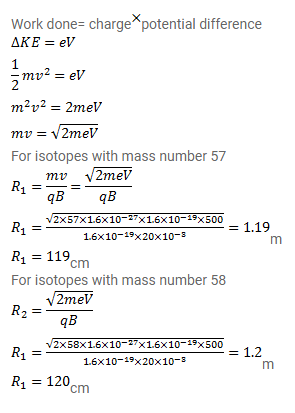# Fe+ ions are accelerated through a potential difference of 500V

Question:

$\mathrm{Fe}^{+}$ions are accelerated through a potential difference of $500 \mathrm{~V}$ and are injected normally into a homogeneous magnetic field $\mathrm{B}$ of strength $20.0 \mathrm{mT}$. Find the radius of the circular paths followed by the isotopes with mass number 57 and 58 . Take the mass of an ion $=A\left(1.6 \times 10^{-27}\right)_{\mathrm{kg}}$ where $\mathrm{A}$ is the mass number.

Solution: